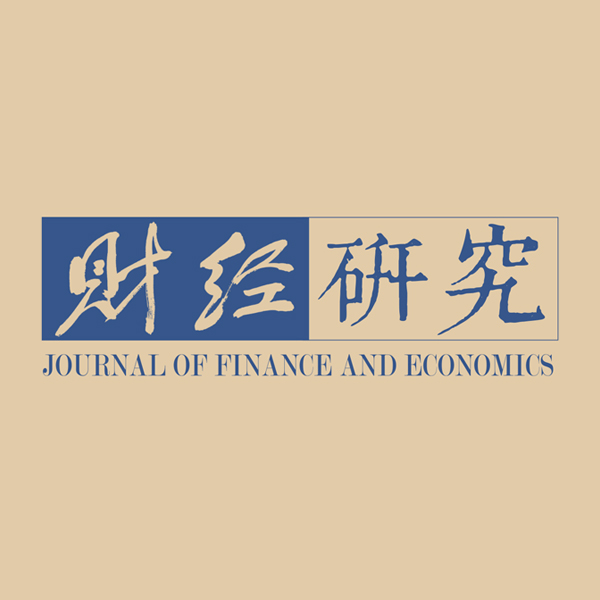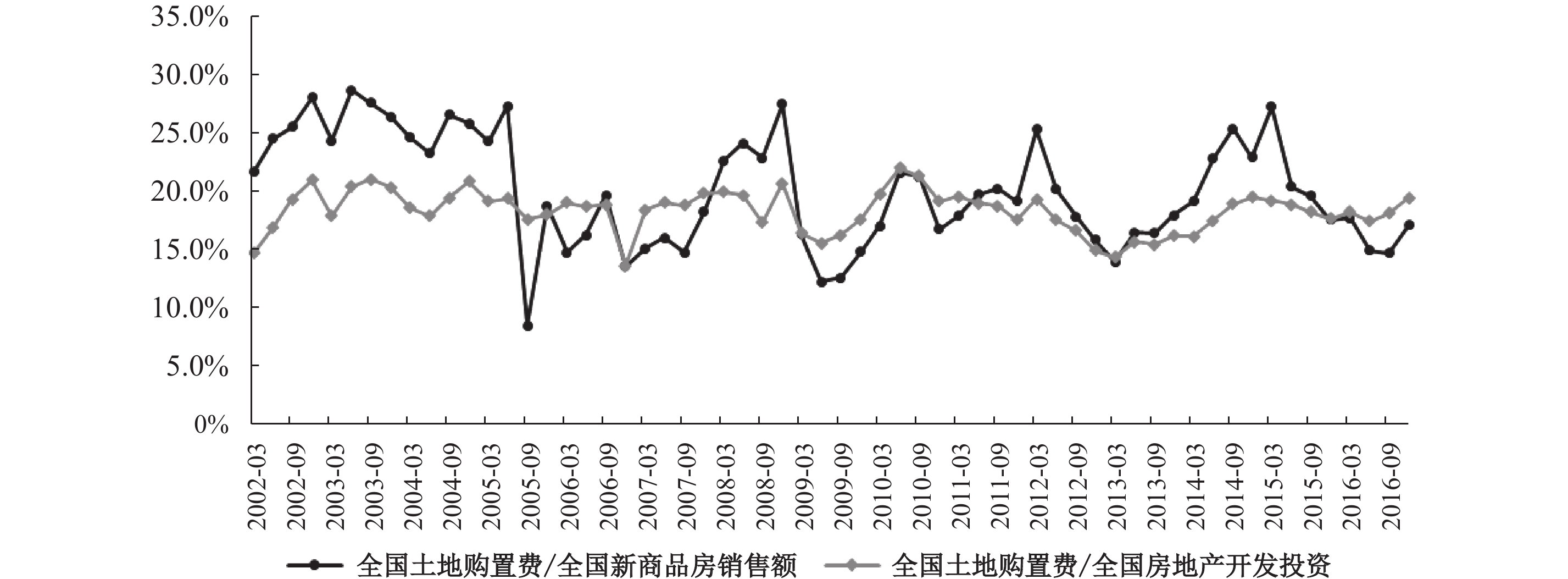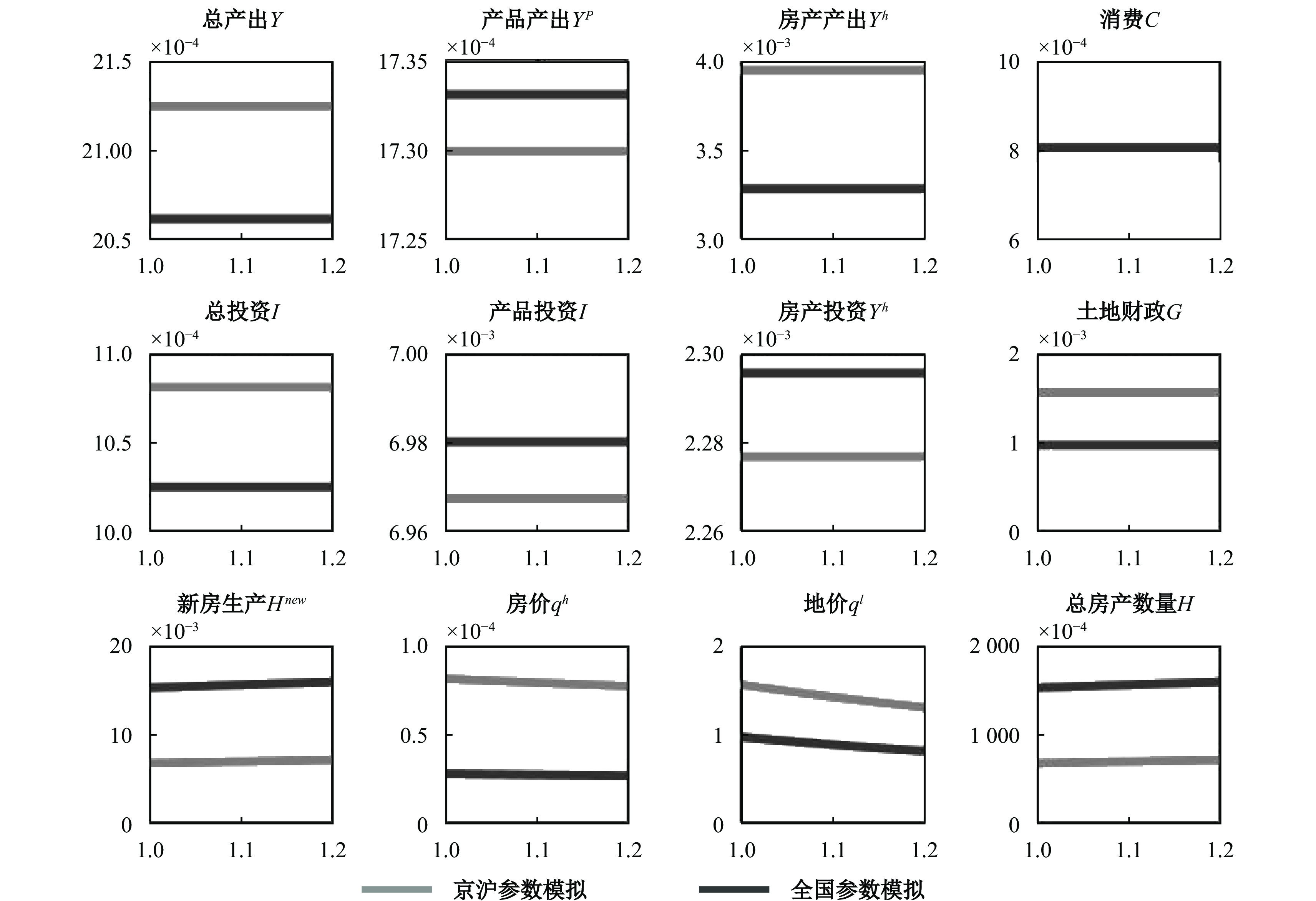﻿ 中国新房总量生产函数与土地供给政策变化效应《财经研究》
2020第46卷第5期

1. 上海对外经贸大学 金融管理学院，上海 201620;
2. 上海交通大学 安泰经济与管理学院，上海 200030

Chinese New Housing’s Aggregate Production Function and the Effect of Land Supply Policy Change
Liu Jianfeng1, Pan Yingli2
1. School of Finance，Shanghai University of International Business and Economics，Shanghai 201620，China;
2. Antai College of Economics and Management，Shanghai Jiao Tong University，Shanghai 200030，China
Summary: China’s land supply system, household’s housing purchasing preference and new housing’s production function are totally different from those in Europe and the United States. As a starting point to study China’s real estate economy from a macro perspective, there is an urgent need to use China’s data to calibrate or estimate a new housing’s aggregate production function with more credible parameters. Therefore, based on a dynamic stochastic general equilibrium (DSGE) model with two production sectors, this paper attempts to do this work. Because we do not pay attention to the short-term fluctuations of China’s macro economy and real estate economy, it is not necessary to establish a large and complex DSGE model that takes into account the monetary and credit financial sectors and various nominal frictions when calibrating or estimating these structural parameters. To this end, this paper follows a common practice of estimating the aggregate production function at home and abroad to construct a fundamental model that does not include these financial and nominal friction factors. The small-scale model in this paper mainly includes six sectors: new housing production, general product production, households, investment products, land resellers and governments. The fundamental model’s estimation finds that in China’s new housing production costs, the shares of land, physical capital and labor are 23%, 67% and 10%, respectively. Obviously, this result is hugely different from Iacoviello and Stefano (2010) about the shares of capital, land and labor of the new housing production function in the United States at approximately 20%, 10% and 70%, respectively. Therefore, we cannot directly borrow the structural parameters in the new housing production function from those countries such as the United States and Europe. In comparison, in the new housing production costs of the first-tier cities such as Beijing and Shanghai, the land’s share is close to 30%, the physical capital’s is slightly over 50%, and the labor’s is 20%. Because capital is basically free to flow in the domestic, the prices of land and labor in the first-tier cities such as Beijing and Shanghai are more expensive than in the other cities, it is reasonable to estimate that the shares of land and labor in the new housing production in the first-tier cities are higher. In addition, Chinese households’ preference parameter for real estate in the first-tier cities such as Beijing and Shanghai is about 1.09, which is significantly larger than the national average of about 0.84. If the model’s setting is basically correct, there may be systematic deviations in the statistics of the Chinese real estate sector, and it is more severe in the national level than in the first-tier cities such as Beijing and Shanghai. The preliminary estimation results show that the sales of new commercial housing in the national level and the first-tier cities such as Beijing and Shanghai are systematically underreported by approximately 8% and 3%, respectively; the land purchasing fees are underreported by about 23% and 6%, respectively; the real estate development investment is overstated by approximately 28% and 23%, respectively. Based on the simulation analysis of the fundamental model, this paper emphasizes that, for the current land supply, local governments should “implement a different policy for those different level cities”: for the third- and fourth-tier cities, they should properly reduce land supply to stabilize land and housing prices in the short-term; for the first- and second-tier cities, they should try to increase land supply to reduce land and housing’ prices in the long-term. On March 12, 2020, the State Council issued the “Decision on Authorization and Entrusted Land Approval Rights” to further deepen the reform of “simplifying administration and decentralization, combining decentralization and management, and optimizing services”, and gave provincial governments greater land autonomy. Obviously, for local governments, this is more conducive to “implement a different land supply policy for those different level cities”, which is demonstrated by this paper. The research method’s contribution is that this paper provides a method for DSGE model’s parameter calibration and Bayesian structure estimation in the case of statistical systematic deviations. This fills the gaps in relevant literature at home and abroad, so it can also be used to study the macroeconomic issues under DSGE framework in the countries with poor statistical data.
Key words: new housing’s aggregate production function    real estate sector’s statistical data quality    land policies    supply-side structural reforms图 1 中国房地产部门新商品房销售额占全国GDP的比重 资料来源：Wind资讯。

2008年美国房地产业次贷危机所引发的全球金融危机给国内外宏观经济学家建模的一个主要教训是，在研究宏观经济波动时，不能忽视货币信贷金融部门以及在新凯恩斯主义模型中已确认的各种名义摩擦因素。最基本的做法是在异质性新房部门和产品生产部门中，或者在异质性家庭部门中引入货币与信贷等金融约束，考察商业银行或金融市场与这些异质性部门之间的互动关系（Liu等，2013；Piazzesi和Schneider，2016；Chen和Wen，2017；Miao和Wang，2018；高然和龚六堂，2017；赵扶扬等，2017；梅冬州等，2018），从而刻画出货币与信贷等金融因素是怎样在经济繁荣时期因放大效应而导致经济过热，或是怎样在经济萧条时期因收缩效应而导致经济过冷，甚至引发金融或经济危机。图 2 全国土地购置费与新商品房销售额和房地产开发投资的平均比率 资料来源：Wind资讯。

（一）新房生产部门

$\mathop {\max }\limits_{\{ L_t,K_t^h,N_t^h\} } q_t^hH_t^{new} - r_t^hK_t^h - {w_t}N_t^h - q_t^l{L_t}$
，其中
$q_t^h$

$H_t^{new}$

$K_t^h$

$N_t^h$

$L_t$

$q_t^l$

${w_t}$
$r_t^h$

 $H_t^{new} = {[{(K_t^h)^{{\alpha _h}}}{(N_t^h)^{1 - {\alpha _h}}}]^{1 - \gamma }}L_t^\gamma$ (1)

$\gamma \in (0,1)$

${\alpha _h} \in (0,1)$

${\alpha _h}$
$\gamma$
。鉴于中国的新房生产函数与欧美国家显著不同，结构参数
${\alpha _h}$
$\gamma$

$\{ K_t^h,N_t^h,L_t\}$

 ${{r_t^h} / {{w_t}}}{\rm{ = [}}{{{\alpha _h}} / {(1 - {\alpha _h})}}]{{N_t^h} / {K_t^h}}$ (2)
 ${{q_t^l} / {{w_t}}} = [{\gamma / {(1 - {\alpha _h})(1 - \gamma )}}]{{N_t^h} / {L_t}}$ (3)

 $q_t^hH_t^{new} = r_t^hK_t^h + {w_t}N_t^h + q_t^lL_t$ (4)

${\delta _h}$

 $H_t^{new} = H_{t + 1} - (1 - {\delta _h})H_t$ (5)

（二）一般产品生产部门

$N_t^p$

$K_t^p$

 $Y_t^p = {A_t}{(K_t^p)^\alpha }{(N_t^p)^{1 - \alpha }}$ (6)

${A_t}$

${A_t}$

 $\log ({{{A_t}} / {{A_{ss}}}}) = {\rho _A}\log ({{{A_{t - 1}}} / {{A_{ss}}}}) - {\varepsilon _{At}},{\rm{ }}{\varepsilon _{At}} \sim {N(0,\sigma _A^2)}$ (7)

${\varepsilon _{At}}$

${A_{ss}}$

$Y_t^p - {r_t}K_t^p - {w_t}N_t^p$
，其中
${w_t}$

${r_t}$

$\{ K_t^p,N_t^p\}$

 ${w_t} = (1 - \alpha ){{Y_t^p} / {N_t^p}}$ (8)
 ${r_t} = \alpha {{Y_t^p} / {K_t^p}}$ (9)

（三）家庭部门

${C_t}$
、房产
${H_{t + 1}}$

${N_t}$

$\mathop {\max }\limits_{\left\{ {{C_t},{H_{t + 1}},{N_t},K_{t + 1}^p,K_{t + 1}^h} \right\}} {E_0}\sum\limits_{t = 0}^\infty {{\beta ^t}(\log {C_t} }+ { {a_{ht}}\log {H_{t + 1}}- {a_n}{N_t}) }$
，其中
$\beta$

${a_{ht}}$

${a_n}$

${a_{ht}}$

 $\log ({{{a_{ht}}}/ {{a_h}}}) = {\rho _{{a_h}}}\log ({{{a_{ht - 1}}} / {{a_h}}}) + {\varepsilon _{a_{ht}}},{\rm{ }}{\varepsilon _{{a_{ht}}}} \sim {N(0,\sigma _{{a_h}}^2)}$ (10)

${\varepsilon _{a_{ht}}}$

${a_h}$

 ${C_t} + q_t^hH_t^{new} + Q_t^pI_t^p + Q_t^hI_t^h = {w_t}{N_t} + {r_t}K_t^p + r_t^hK_t^h + {G_t}$ (11)

$I_t^p$

$I_t^h$

$Q_t^p$
$Q_t^h$

${G_t}$

${G_t}$
。为使问题简化，本文假设政府并不直接执行“土地财政”开支
${G_t}$
，而是将其完全转移支付给家庭部门，由家庭部门消费和投资并购买商品房。

$\delta$

 $I_t^p = K_{t + 1}^p - (1 - \delta )K_t^p$ (12)
 $I_t^h = K_{t + 1}^h - (1 - \delta )K_t^h$ (13)

${C_t}$
、新购买住房
$H_t^{new}$

$I_t^h$
$I_t^p$
），等式右边的当期总收入是劳动收入（
${w_t}{N_t}$
）、在新房生产部门和一般产品生产部门持有的总资本收益（
$r_tK_t^p + r_t^hK_t^h$
）以及来自政府转移支付的收入
${G_t}$

${\lambda _t}$
，则
$\{ {N_t},K_{t + 1}^p,K_{t + 1}^h,{C_t},{H_{t + 1}}\}$

 ${a_n} = {w_t}{\lambda _t}$ (14)
 $Q_t^p{\lambda _t} = \beta {{\rm{E}}_t}[{r_{t + 1}}{\lambda _{t + 1}} + (1 - \delta )Q_{t + 1}^p{\lambda _{t + 1}}]$ (15)
 $Q_t^h{\lambda _t} = \beta {{\rm{E}}_t}[r_{t + 1}^h{\lambda _{t + 1}} + (1 - \delta )Q_{t + 1}^h{\lambda _{t + 1}}]$ (16)
 ${1 / {{C_t}}} = {\lambda _t}$ (17)
 $q_t^h{\lambda _t} - \beta (1 - {\delta _h}){{\rm{E}}_t}(q_{t + 1}^h{\lambda _{t + 1}}) = {{{a_{ht}}} / {{H_{t + 1}}}}$ (18)

（四）实物性投资品厂商

$Q_t^p$
$Q_t^h$

$I_t^p$
$I_t^h$

$\mathop {\max }\limits_{\{ I_t^p,I_t^h\} } {E_0}\sum\limits_{t = 0}^\infty {{\beta ^t}\dfrac{{{\lambda _t}}}{{{\lambda _0}}}\{ Q_t^pI_t^p - }$
$[1 + \dfrac{{{\omega _p}}}{2}{{(\dfrac{{I_t^p}}{{I_{t - 1}^p}} - 1)^2}}]\dfrac{{I_t^p}}{{{Z_t}}} {+ Q_t^hI_t^h - [1 + \dfrac{{{\omega _h}}}{2}{{(\dfrac{{I_t^h}}{{I_{t - 1}^h}} - 1)^2}}]I_t^h\} }$
，其中
${\omega _p} \geqslant 0$
${\omega _h} \geqslant 0$

${Z_t}$

${Z_t}$

 $\log ({{{Z_t}} / {{Z_{ss}}}}) = {\rho _Z}\log ({{{Z_{t - 1}}} / {{Z_{ss}}}}) - {\varepsilon _{Zt}},{\rm{ }}{\varepsilon _{Zt}} \sim {N(0,\sigma _Z^2)}$ (19)

${\varepsilon _{Zt}}$

${Z_{ss}}$

$I_t^p$
$I_t^h$

 $Q_t^p = 1 + \frac{{{\omega _p}}}{2}{(\frac{{I_t^p}}{{I_{t - 1}^p}} - 1)^2}\frac{1}{{{Z_t}}} + {\omega _p}(\frac{{I_t^p}}{{I_{t - 1}^p}} - 1)\frac{{I_t^p}}{{I_{t - 1}^p}}\frac{1}{{{Z_t}}} - \beta {\omega _p}{{\rm{E}}_{\rm{t}}}\frac{{{\lambda _{t + 1}}}}{{{\lambda _t}}}(\frac{{I_{t + 1}^p}}{{I_t^p}} - 1){(\frac{{I_{t + 1}^p}}{{I_t^p}})^2}\frac{1}{{{Z_{t + 1}}}}$ (20)
 $Q_t^h = 1 + \frac{{{\omega _h}}}{2}{(\frac{{I_t^h}}{{I_{t - 1}^h}} - 1)^2} + {\omega _h}(\frac{{I_t^h}}{{I_{t - 1}^h}} - 1)\frac{{I_t^h}}{{I_{t - 1}^h}} - \beta {\omega _h}{{\rm{E}}_{\rm{t}}}\frac{{{\lambda _{t + 1}}}}{{{\lambda _t}}}(\frac{{I_{t + 1}^h}}{{I_t^h}} - 1){(\frac{{I_{t + 1}^h}}{{I_t^h}})^2}$ (21)

$Q_t^p$

$I_t^p$

$Q_t^h$

$I_t^h$

（五）土地转卖商

$q_t^l$

${L_t}$

$\mathop {\max }\limits_{\{ {L_t}\} } {E_0}\sum\limits_{t = 0}^\infty {{\beta ^t}\dfrac{{{\lambda _t}}}{{{\lambda _0}}}\{ q_t^l{L_t} - [1 + \dfrac{{{\omega _l}}}{2}{{(\dfrac{{{L_t}}}{{{L_{t - 1}}}} - 1)^2}}]Q_t^l{L_t}\} }$
，其中
${\omega _l} \geqslant 0$

$Q_t^l$

${L_t}$

 $q_t^l = [1 + \frac{{{\omega _l}}}{2}{(\frac{{{L_t}}}{{{L_{t - 1}}}} - 1)^2} + {\omega _l}(\frac{{{L_t}}}{{{L_{t - 1}}}} - 1)\frac{{{L_t}}}{{{L_{t - 1}}}}]Q_t^l - \beta {\omega _l}{{\rm{E}}_{\rm{t}}}\frac{{{\lambda _{t + 1}}}}{{{\lambda _t}}}(\frac{{{L_{t + 1}}}}{{{L_t}}} - 1){(\frac{{{L_{t + 1}}}}{{{L_t}}})^2}]Q_{t + 1}^l$ (22)

（六）政府部门

${L_t}$

 $\log ({{{L_t}}/ {\bar L}}) = {\rho _L}\log ({{{L_{t - 1}}} / {\bar L}}) - {\varepsilon _{Lt}},{\rm{ }}{\varepsilon _{Lt}} \sim {N(0,\sigma _L^2)}$ (23)

${\varepsilon _{Lt}}$

$\bar L$

$Q_t^l$
，所以政府部门的土地出售收入为
$Q_t^l{L_t}$
。假设不考虑政府的其他财政收入，
${G_t}$

 ${G_t} = Q_t^lL_t$ (24)

（七）一般均衡与经济动力系统

 ${C_t} + q_t^hH_t^{new} + Q_t^pI_t^p + Q_t^hI_t^h = {Y_t} + Q_t^lL_t$ (25)

${Y_t}$

 ${Y_t} = Y_t^h + Y_t^p$ (26)

$Y_t^h$

 $Y_t^h = r_t^hK_t^h + {w_t}N_t^h$ (27)

$V_t^{hnew}$

 $V_t^{hnew} = q_t^hH_t^{new} = r_t^hK_t^h + {w_t}N_t^h + q_t^lL_t$ (28)

${N_t}$

 ${N_t} = N_t^h + N_t^p$ (29)

${I_t}$

 ${I_t} = I_t^p + I_t^{hd}$ (30)

$I_t^{hd}$

$I_t^l$

 $I_t^{hd} = I_t^h + I_t^l$ (31)
 $I_t^l = Q_t^l{L_t}$ (32)

${K_t}$

$V_{t + 1}^h$

 ${K_t} = K_t^h + K_t^p$ (33)
 $V_{t + 1}^h = q_t^hH_{t + 1}$ (34)

（一）数据描述

（二）参数校准

$\{ {\omega _p},{\omega _h},{\omega _l}\}$
、其他不涉及房地产部门的6个标准结构参数
$\{ {a_n},\beta ,\alpha ,\delta ,{A_{ss}},{Z_{ss}}\}$
、其他涉及房地产部门的5个结构参数
$\{ {\delta _h},\gamma ,{\alpha _h},{a_h},\bar L\}$

$\{ {\rho _A},{\sigma _A},{\rho _Z},{\sigma _Z},{\rho _{{a_h}}},{\sigma _{{a_h}}},{\rho _L},{\sigma _L}\}$
。参数校准过程（受篇幅限制未报告）包括：冲击相关参数和非房地产部门相关参数的校准、房地产部门相关参数的校准方法、中国房地产部门相关参数初次校准的失败及原因分析以及引入额外信息后对中国房地产部门相关参数的成功试校准。初次校准失败的原因可以从两个方面来分析与评判：第一，本文的模型设定存在严重错误；第二，用于校准的数据可能存在统计偏差。一方面，房地产部门的生产函数、一般产品部门的生产函数以及家庭部门的效用函数都来自国内外文献中的标准设置，所以不能认为模型存在明显的设定错误。另一方面，由于主观折现因子
$\beta$

$\delta$

${x_2}$

${x_1} = \gamma$

${x_2}$
${x_1}$

 参数符号 参数名称 校准值 校准依据 ${a_n}$ 闲暇负效用常数 1 标准化为1 $\beta$ 主观折现因子 0.994 2002Q1−2016Q4的一年期存款年平均利率为2.56% $\alpha$ 中间产品生产资本份额 0.50 Chow和Li（2002）、Song等（2011）等 $\delta$ 实物性资产折旧率 0.025 国外标准文献 ${A_{ss}}$ 稳态时的全要素生产率 1 标准化为1 ${Z_{ss}}$ 稳态时的投资专用技术 1 标准化为1 ${\omega _p}$ 一般产品生产部门的投资调节成本常数 0.18 Liu等（2013） ${\omega _h}$ 新房生产部门的投资调节成本常数 0.18 Liu等（2013） ${\omega _l}$ 土地转卖中的调节成本常数 0.18 Liu等（2013） ${\delta _h}$ 房产折旧率 0.01 Iacoviello和Stefano（2010） $\bar L$ 土地供给常数 1 标准化为1 $\gamma$ 全国新房生产中的土地份额 0.211 2016Q1−Q4全国和京沪两地的土地购置费与新商品房销售额的加权平均比值① ${\alpha _h}$ 全国新房生产中与资本份额相关的参数 0.884 2016Q1−Q4全国和京沪两地的土地购置费与房地产开发投资的加权平均比值 ${a_h}$ 全国的家庭房产需求偏好强度 0.818 2016Q1−Q4全国和京沪两地的新商品房销售额与全国总产出的加权平均比值 ${\gamma ^{JH}}$ 京沪两地新房生产中的土地份额 0.284 2016Q1−Q4京沪两地的土地购置费与新商品房销售额的平均比值 $\alpha _h^{JH}$ 京沪两地新房生产中与资本份额相关的参数 0.715 2016Q1−Q4京沪两地的土地购置费与房地产开发投资的平均比值 $a_h^{JH}$ 购买京沪两地新房的家庭房产需求偏好强度 1.084 2016Q1−Q4京沪两地的新商品房销售额与全国总产出的平均比值

（一）系统性偏差数据的处理

$b_t^r$
，真值为
$b_t^t$
，以
$x_t^b = {{b_t^r}/ {b_t^t}}$

$b_t$
，以
$X_t^b = {{b_t^t} / {b_t}}$

 ${{b_t^r} / {b_t}} = x_t^bX_t^b$ (35)

${{b_t^r}/ {b_{t - 1}^r}} = {g_{b_t^r}}$
${{x_t^b} / {x_{t - 1}^b}} = {g_{x_{}^b}}{g_{x_t^b}},{\rm{ }}{g_{x_t^b}}\sim N(1,\sigma _{{g_{x_{}^b}}}^2)$
${{b_t} / {b_{t - 1}}} = {g_{{b_t}}}$
${{X_t^b} / {X_{t - 1}^b}} = {g_{X_{}^b}}{g_{X_t^b}},\;{g_{X_t^b}}\sim$
$N(1,\sigma _{{g_{X_{}^b}}}^2)$
，则有：

 ${{{g_{b_t^r}}}/ {{g_{{b_t}}}}} = ({g_{x_{}^b}}{g_{X_{}^b}})({g_{x_t^b}}{g_{X_t^b}})$ (36)

${g_{x_{}^b}} \ne 1$

${\tilde g_{b_t^r}} = {\rm{log(}}{g_{b_t^r}}) - {\rm{log(}}{g_{{b^r}}})$
${\tilde g_{{b_t}}} = {\rm{log(}}{g_{{b_t}}}) - {\rm{log(}}{g_b}) = {\rm{log(}}{g_{{b_t}}})$
，其中
${g_{{b^r}}}$
${g_b} = 1$

${g_{b_t^r}}$
${g_{{b_t}}}$

 ${\tilde g_{b_t^r}} = {\tilde g_{{b_t}}} + \varepsilon _{{b_t}},{\rm{ }}\varepsilon _{{b_t}} = \log ({g_{x_t^b}}{g_{X_t^b}})\sim N(0,\sigma _{{g_b}}^2)$ (37)

${\varepsilon _{{b_t}}}$

 ${{\tilde g}}_{{b}_{t}^{r}} = {{\tilde g}}_{{b}_{t}} + {{\varepsilon }}_{{b}_{t}}$ (38)

${{\tilde g}}_{{b}_{t}^{r}} = {\left( {\begin{array}{*{20}{c}} {{{\tilde g}_{I_t^{rl}}}}&{{{\tilde g}_{V_t^{rhnew}}}}&{{{\tilde g}_{I_t^{rhd}}}} \end{array}} \right)^\prime }$
${{\tilde g}}_{{b}_{t}} = {\left( {\begin{array}{*{20}{c}} {{{\tilde g}_{I_t^l}}}&{{{\tilde g}_{V_t^{hnew}}}}&{{{\tilde g}_{I_t^{hd}}}} \end{array}} \right)^\prime }$
${{\varepsilon }}_{{b}_{t}} = {\left( {\begin{array}{*{20}{c}} {{\varepsilon _{I_t^l}}}&{{\varepsilon _{V_t^{hnew}}}}&{{\varepsilon _{I_t^{hd}}}} \end{array}} \right)^\prime }$

 ${\tilde g_{Y_t^r}} = {\tilde g_{Y_t}}$ (39)
 ${\tilde g_{C_t^r}} = {\tilde g_{C_t}}$ (40)

（二）房地产部门统计数据季度增长的描述性统计

 土地购置费季度增长 新商品房销售额季度增长 房地产开发投资季度增长 产出季度增长 消费季度增长 全国 均值 0.0449 0.0488 0.0402 0.0225 0.0233 标准差 0.1147 0.2118 0.0427 0.0065 0.0084 京沪两地 均值 0.0420 0.0300 0.0218 0.0204 − 标准差 0.3156 0.2145 0.1126 0.0498 − 资料来源：Wind资讯。

（三）房地产部门结构参数的贝叶斯估计

$\{ \gamma ,{\alpha _h},{a_h}\}$
。首先，需要根据上文中的试校准结果慎重选取参数的先验分布。对于全国新房生产中的土地份额
$\gamma$

${\alpha _h}$
，设定为均值分别为0.211和0.884、标准差都为0.02，且取值范围都在区间（0，1）内的Beta分布；对于全国的家庭房产需求偏好强度
${a_h}$
，设定为均值为0.818、标准差为0.08的Gamma分布。对于投资调节成本参数
$\{ {\omega _p},{\omega _h},{\omega _l}\}$
，根据Liu等（2013）的研究，设定为均值为0.18、标准差为0.08的Gamma分布。对于外生冲击参数
$\{ {\rho _L},{\sigma _L},{\rho _{{a_h}}},{\sigma _{{a_h}}},{\rho _A},{\sigma _A},{\rho _z},{\sigma _z}\}$
，根据An和Schorfheide（2007）的研究，假设AR（1）系数
$\{ {\rho _L},{\rho _{{a_h}}},{\rho _A},{\rho _z}\}$

$\{ {\sigma _L},{\sigma _{{a_h}}},{\sigma _A},{\sigma _z}\}$

$\{ {\sigma _{{g_{I_t^l}}}},{\sigma _{{g_{V_t^{hnew}}}}},{\sigma _{{g_{I_t^{hd}}}}}\}$

$\{ \gamma ,{\alpha _h},{a_h}\}$

$\gamma$
、资本份额
${\alpha _h}(1 - \gamma )$

$(1 - {\alpha _h})(1 - \gamma )$

$\{ {\omega _p},{\omega _h},{\omega _l}\}$

$\{ {\gamma ^{JH}},\alpha _h^{JH},a_h^{JH}\}$

${\gamma ^{JH}}$

$\alpha _h^{JH}(1 - {\gamma ^{JH}})$

$(1 - \alpha _h^{JH})(1 - {\gamma ^{JH}})$

 待估参数 先验分布 先验均值 众数 后验均值与90%的置信区间 $\gamma$ Beta 0.211 0. 2207 0.2289 [0.1953，0.2663] ${\alpha _h}$ Beta 0.884 0.8805 0.8680 [0.8312，0.9052] ${a_h}$ Gamma 0.818 0.8087 0.8426 [0.7123，0.9818] ${\omega _p}$ Gamma 0.18 0.3046 0.2707 [0.1233，0.4162] ${\omega _h}$ Gamma 0.18 0.5070 0.9383 [0.5562，1.2170] ${\omega _l}$ Gamma 0.18 0.1766 0.1875 [0.0579，0.3103] ${\rho _L}$ Beta 0.90 0.9506 0.9128 [0.8763，0.9380] ${\rho _{{a_h}}}$ Beta 0.90 0.9046 0.9011 [0.8683，0.9339] ${\rho _A}$ Beta 0.90 0.9408 0.9313 [0.9006，0.9584] ${\rho _Z}$ Beta 0.90 0.8737 0.8569 [0.8174，0.8952] ${\sigma _L}$ Inverse Gamma 0.01 0.1129 0.0350 [0.0021，0.1113] ${\sigma _{{a_h}}}$ Inverse Gamma 0.01 0.0046 0.0084 [0.0025，0.0155] ${\sigma _A}$ Inverse Gamma 0.01 0.0068 0.0056 [0.0043，0.0071] ${\sigma _Z}$ Inverse Gamma 0.01 0.0102 0.0138 [0.0096，0.0177] ${\sigma _{{g_{{I^l}}}}}$ Inverse Gamma 0.01 0.1092 0.1135 [0.0956，0.1305] ${\sigma _{{g_{{V^{hnew}}}}}}$ Inverse Gamma 0.01 0.2071 0.2145 [0.1817，0.2480] ${\sigma _{{g_{{I^{hd}}}}}}$ Inverse Gamma 0.01 0.0351 0.0547 [0.0351，0.0726]

（四）方差分解：各种冲击的重要性

 土地供给政策冲击 房产需求偏好冲击 全要素生产率冲击 投资专用技术冲击 总产出 4.08（0.02） 0.10（0.01） 70.96（99.76） 24.86（0.21） 房产产出 39.19（6.99） 0.92（1.88） 2.44（57.95） 57.45（33.18） 产品产出 0.46（0.00） 0.01（0.00） 61.10（99.54） 38.43（0.45） 消费 0.25（0.02） 0.01（0.01） 6.34（76.98） 93.41（22.98） 房产投资 2.08（0.37） 0.04（0.11） 1.48（61.31） 96.40（38.22） 产品投资 0.37（0.02） 0.01（0.01） 10.72（94.06） 88.90（5.91） 土地财政 34.76（5.58） 0.99（1.91） 2.62（58.83） 61.63（33.69） 新房生产 45.59（6.66） 0.02（0.12） 1.38（63.98） 53.01（29.24） 房价 1.37（0.20） 1.67（3.16） 3.30（64.21） 93.67（32.43） 地价 89.50（26.57） 0.16（1.48） 0.42（45.75） 9.91（26.20） 注：括号内外的数值分别对应于京沪两地和全国的参数模拟和估计结果，方差分解结果用百分数表示。

（一）土地供给增减的宏观经济效应

1. 传导机制的理论分析

${L_t}$

${\varepsilon _{Lt}}$

$Q_t^l{L_t}$

${G_t}$

$H_t^{new}$

$I_t^h$

${H_t}$

$Y_t^h$

$q_t^l$

$q_t^h$

$I_t^p$

${C_t}$

$Y_t^p$

${G_t}$
，而是将其完全转移支付给家庭部门，由家庭部门消费和投资并购买商品房。由上文模型中家庭部门的设置可知，家庭预算约束式（11）右边的政府转移支付收入增加，左边在房地产部门的实物性投资
$I_t^h$

$I_t^p$

$Y_t^h$

$Y_t^p$

${C_t}$

${Y_t}$

${Y_t}$

2. 短期效应图 3 政府土地供给增减的脉冲反应

3. 长期效应

（二）一二线城市土地供给政策变化的长效机制

（三）三四线城市土地供给政策变化的短期效果图 4 政府土地供给增加的长期宏观经济效应

* 作者感谢上海交通大学安泰经济与管理学院许志伟老师和两位匿名审稿人的建议性意见！当然，文责自负。

① 由于研究目的不同，国内有关房地产经济的其他宏观文献大多没有设定标准新房总量生产函数。例如，蔡明超等（2011）以及陈彦斌和邱哲圣（2011）的研究中没有房地产部门生产函数；侯成琪和龚六堂（2014）在房地产部门生产函数中将土地做了一个类似于全要素生产率的设定，其仅作为土地供给冲击存在；陈彦斌等（2015）设定的房地产部门生产函数中不包含劳动要素。

② 作者每年大约在8月份更新前一年的数据，可以从以下网址下载：http://www.nber.org/data-appendix/c13592/https://www.frbatlanta.org/cqer/research/china-macroeconomy.aspx

③ 仅采用全国数据出现校准失败，推断出全国数据可能存在一定程度上的瞒漏报或虚重报情况，从而引入比较准确的京沪两地数据来修正初始校准值。下同。

  蔡明超, 黄徐星, 赵戴怡. 房地产市场反周期宏观调控政策绩效的微观分析[J]. 经济研究, 2011(S1): 80–89.  陈彦斌, 郭豫媚, 陈伟泽. 2008年金融危机后中国货币数量论失效研究[J]. 经济研究, 2015(4): 21–35.  陈彦斌, 邱哲圣. 高房价如何影响居民储蓄率和财产不平等[J]. 经济研究, 2011(10): 25–38.  高然, 龚六堂. 土地财政、房地产需求冲击与经济波动[J]. 金融研究, 2017(4): 32–45. DOI:10.3969/j.issn.1009-3109.2017.04.005  郭庆旺, 贾俊雪. 中国全要素生产率的估算: 1979−2004[J]. 经济研究, 2005(6): 51–60.  侯成琪, 龚六堂. 货币政策应该对住房价格波动作出反应吗——基于两部门动态随机一般均衡模型的分析[J]. 金融研究, 2014(10): 15–33.  梅冬州, 崔小勇, 吴娱. 房价变动、土地财政与中国经济波动[J]. 经济研究, 2018(1): 35–49.  王云清, 朱启贵, 谈正达. 中国房地产市场波动研究——基于贝叶斯估计的两部门DSGE模型[J]. 金融研究, 2013(3): 101–113.  许志伟, 林仁文. 我国总量生产函数的贝叶斯估计——基于动态随机一般均衡的视角[J]. 世界经济文汇, 2011(2): 87–102. DOI:10.3969/j.issn.0488-6364.2011.02.006  许志伟, 吴化斌. 企业组织资本对中国宏观经济波动的影响[J]. 管理世界, 2012(3): 23–33.  赵扶扬, 王忏, 龚六堂. 土地财政与中国经济波动[J]. 经济研究, 2017(12): 46–61.  周彬, 周彩. 土地财政、企业杠杆率与债务风险[J]. 财贸经济, 2019(3): 19–36.  An S, Schorfheide F. Bayesian analysis of DSGE models[J]. Econometric Reviews, 2007, 26(2−4): 113–172. DOI:10.1080/07474930701220071  Bernanke B S, Gertler M, Gilchrist S. The financial accelerator in a quantitative business cycle framework[A]. Taylor J B, Woodford M. Handbook of macroeconomics （Vol.1）[M]. Amsterdam: Elsevier, 1999.  Chang C, Chen K J, Waggoner D F, et al. Trends and cycles in China’s macroeconomy[A]. Eichenbaum M, Parker J A. NBER Macroeconomics Annual 2015[M]. University of Chicago Press, 2016.  Chen K J, Wen Y. The great housing boom of China[J]. American Economic Journal: Macroeconomics, 2017, 9(2): 73–114. DOI:10.1257/mac.20140234  Chow G C, Li K W. China’s economic growth: 1952-2010[J]. Economic Development and Cultural Change, 2002, 51(1): 247–256. DOI:10.1086/344158  Hansen G D. Indivisible labor and the business cycle[J]. Journal of Monetary Economics, 1985, 16(3): 309–327. DOI:10.1016/0304-3932(85)90039-X  Iacoviello M, Stefano N. Housing market spillovers: Evidence from an estimated DSGE model[J]. American Economic Journal: Macroeconomics, 2010, 2(2): 125–164. DOI:10.1257/mac.2.2.125  Ireland P N. A method for taking models to the data[J]. Journal of Economic Dynamics and Control, 2004, 28(6): 1205–1226. DOI:10.1016/S0165-1889(03)00080-0  Kydland F E, Prescott E C. Time to build and aggregate fluctuations[J]. Econometrica, 1982, 50(6): 1345–1370. DOI:10.2307/1913386  Liu Z, Wang P F, Zha T. Land-price dynamics and macroeconomic fluctuations[J]. Econometrica, 2013, 81(3): 1147–1184. DOI:10.3982/ECTA8994  Miao J J, Wang P F. Asset bubbles and credit constraints[J]. American Economic Review, 2018, 108(9): 2590–2628. DOI:10.1257/aer.20160782  Piazzesi M, Schneider M. Housing and macroeconomics[A]. Taylor J B, Woodford M. Handbook of macroeconomics （Vol.2）[M]. Amsterdam: Elsevier, 2016.  Song Z, Storesletten K, Zilibotti F. Growing like China[J]. The American Economic Review, 2011, 101(1): 196–233. DOI:10.1257/aer.101.1.196  Wei S J, Zhang X B. The competitive saving motive: Evidence from rising sex ratios and savings rates in China[J]. Journal of Political Economy, 2011, 119(3): 511–564. DOI:10.1086/660887